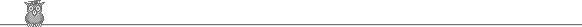### 50th Putnam 1989Problem A2

Let R be the rectangle 0 ≤ x ≤ a, 0 ≤ y, ≤ b. Evaluate ∫R ef(x, y) dx dy, where f(x, y) = max(b2x2, a2y2).

Solution

Divide the rectangle into two by the diagonal from (0, 0) to (a, b). The required value is evidently twice the integral over the lower half of eg(x), where g(x) = b2x2. But integrating over y just gives a factor bx/a, and the integration over x is then trivial to give (ek - 1)/(2ab), where k = a2b2. So the required value is twice this or (ek - 1)/(ab).